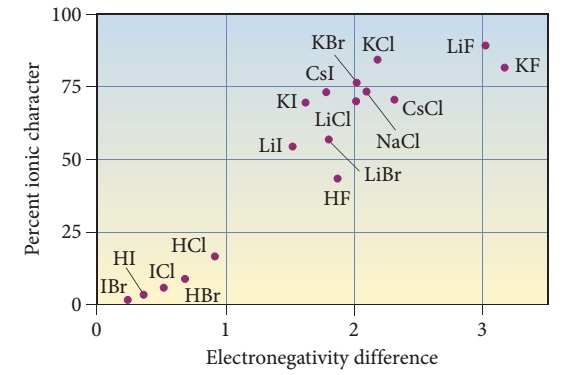# Problem: Estimate the percent ionic character of the CO bond. The electronegativity of C is 2.5 and O is 3.5.

###### FREE Expert Solution

The percent ionic character of a bond can be estimated using the following equation:where ΔEN = electronegativity difference.

92% (387 ratings)###### Problem Details

Estimate the percent ionic character of the CO bond. The electronegativity of C is 2.5 and O is 3.5.